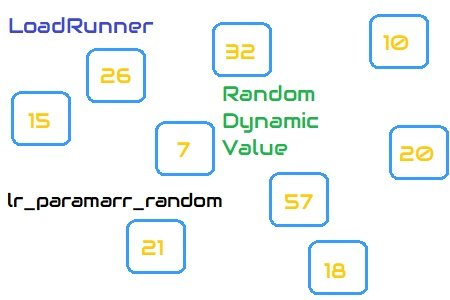# Random Value from Correlation Parameter in LoadRunner## Problem:

How to select a random value from the correlation parameter in LoadRunner?

## Explanation:

Sometimes there is a requirement to pass a random value from a list of dynamic values captured by a correlation function i.e. web_reg_save_param(). Let’s consider a web page has 10 items which could be different for all users. Now, you want to randomly choose 1 item appeared on the web page and place an order. What will be your approach?

Definitely:

1. You will add a correlation function before the request whose response contains the name of items
2. You will give “Ord=All” to capture all the name of all the items
``````web_reg_save_param("c_itemName",
"LB=itemname=\"",
"RB=\"",
"Ord=All",
LAST);``````

Additional Information: When you give “Ord=All” then the correction parameter (c_itemName) acts as an array and captures all the values which satisfy the left and right boundaries. If you want to choose any specific value then you can append “_<number>” in the correlation parameter and pass into the subsequent request. <number> denotes the place of the item appeared on the webpage.
For example, if you need to pick 2nd value then the correlation parameter will be “c_itemName_2”.

## Solution:

To pick the random value from the correlation parameter, you have to use the following statement:

lr_save_string(lr_paramarr_random(“c_itemName”),”randParam”);

where:

lr_paramarr_random: This function returns a random value from the parameter array. The return value will be a ‘String’. In the above example, a random value (string) will be picked from “c_itemName” array.

lr_save_string: This function is used to save a string into a parameter. In the above example, the random value (string) extracted by lr_paramarr_random will be saved in “randParam”.

Now, you can use “randParam” as a parameter in the script which will pass a random value extracted from the list of captured dynamic values.

You may be interested: